The Spheres ClipArt gallery includes 74 images of spheres, including parts and zones of spheres and hemispheres. A sphere is a 3-dimensional figure in which all points are equidistant from a center point.### Symmetrical Spherical Triangles

Diagram showing 2 symmetrical spherical triangles.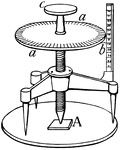### Spherometer

"An instrument for measuring the radii of spheres; a sphere-measurer. It is of especial service to opticians…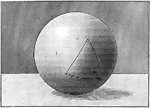### Isosceles Spherical Triangle

Illustration of an isosceles spherical triangle.### Isosceles Spherical Triangle

Illustration of an isosceles spherical triangle.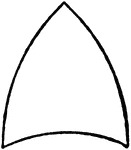### Spherical Triangle

Diagram showing a spherical triangle.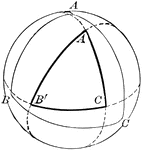### Spherical Triangle

Diagram showing a spherical triangle constructed by the intersection of polar arcs.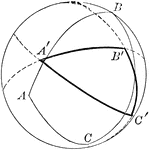### Spherical Triangle

Diagram showing a spherical triangle constructed by the intersection of polar arcs.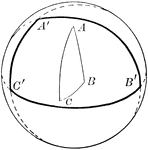### Spherical Triangle

Diagram showing a spherical triangle constructed by the intersection of polar arcs.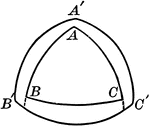### Polar and Spherical Triangles

Illustration of polar and spherical triangles.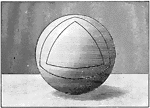### Polar Triangles in Sphere

Illustration of polar triangles.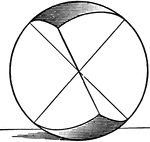### Symmetric Spherical Triangles

Illustration of symmetrical spherical triangles.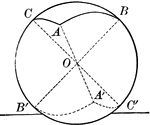### Symmetric Spherical Triangles

Illustration of symmetrical spherical triangles.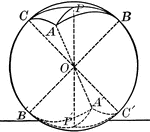### Symmetric Spherical Triangles

Illustration of symmetrical spherical triangles.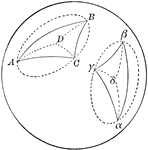### Two Symmetrical Spherical Triangles

Diagram showing a 2 symmetrical spherical triangles.